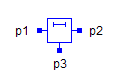Multiphase Constant Current - MapleSim Help

Multiphase Constant Current

Multiphase constant current sourceDescription The Multiphase Constant Current (or Constant Current) component contains $m$ single-phase constant current source models, each connected between corresponding phases of ${\mathrm{plug}}_{p}$ and ${\mathrm{plug}}_{n}$, with the currents set by the vector parameter $I$.Connections

 Name Description Modelica ID ${\mathrm{plug}}_{p}$ Positive $m$-phase plug plug_p ${\mathrm{plug}}_{n}$ Negative $m$-phase plug plug_nParameters

 Name Default Units Description Modelica ID $m$ $3$ Number of phases m $I$ $\mathrm{fill}\left(1,m\right)$ $A$ Vector of currents IModelica Standard Library The component described in this topic is from the Modelica Standard Library. To view the original documentation, which includes author and copyright information, click here.